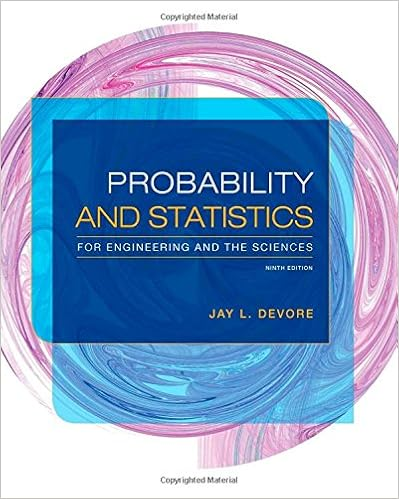# For each of the following questions say whether the

• Homework Help
• 3
• 100% (3) 3 out of 3 people found this document helpful

This preview shows page 2 - 3 out of 3 pages.

##### We have textbook solutions for you!
The document you are viewing contains questions related to this textbook.The document you are viewing contains questions related to this textbook.
Chapter 3 / Exercise 88
Probability and Statistics for Engineering and the Sciences
DevoreExpert Verified
3.For each of the following questions, say whether the random process is a binomial process or not, and explainyour answer. As part of your explanation, you will want to comment on the potential validity of each of thefour things that must be true for a process to be a binomial process.1Stat 371Spring 2017(a)One basketball player attempts 10 free throws and the number of successful attempts is totalled.
(b)Ten different basketball players each attempt 1 free throw and the total number of successful attemptsistotalled.
4.Let B Bin(20,0.2). Compute the following probabilities. I would suggest computing these with a handcalculator using the formula provided in class (you will not have R on the exams!), but you can check youranswers using R if you wish.(a)P(B = 4).
(b)P(B ≤ 1).
1).
##### We have textbook solutions for you!
The document you are viewing contains questions related to this textbook.The document you are viewing contains questions related to this textbook.
Chapter 3 / Exercise 88
Probability and Statistics for Engineering and the Sciences
DevoreExpert Verified
R Notes• See the file, ’RVs and Dists R Code.txt’ on Learn for how to compute binomial and normal probabilities using R.2
•••